# 0.展示PTA总分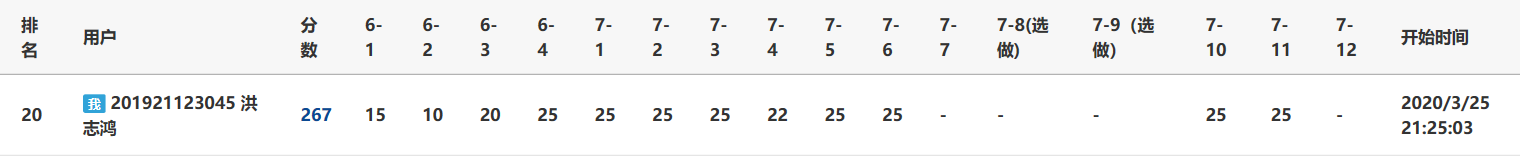# 1.本章学习总结

## 串的BF\KMP算法（功能：找到主串中子串第一次出现的位置）

### BF算法

定义i和j分别表示主串和子串中字符的下标
while i<主串长度且j<子串长度
if i所表示的主串中的字符与j所表示的子串字符相等
下标i和j分别自增1
end if
else
重新开始新的匹配，i从初始匹配的下一个开始，即i=i-j+1;
j从初始位置开始，即j=0；
end else
end while
if j>=子串长度
返回初始位置即i-子串长度
end if
else
说明未找到，返回对应数字表示寻找失败
end else


## kmp

### 具体代码

int KMPIndex(SqString s,SqString t)
{     int next[MaxSize], i=0, j=0;
GetNext(t,next);
while (i<s.length && j<t.length)
{
if (j==-1 || s.data[i]==t.data[j])
{    i++;
j++;			//i、j各增1
}
else   j=next[j]; 		//i不变，j后退
}
if (j>=t.length)
return(i-t.length);		//返回匹配模式串的首字符下标
else
return(-1);		//返回不匹配标志
}



### next数组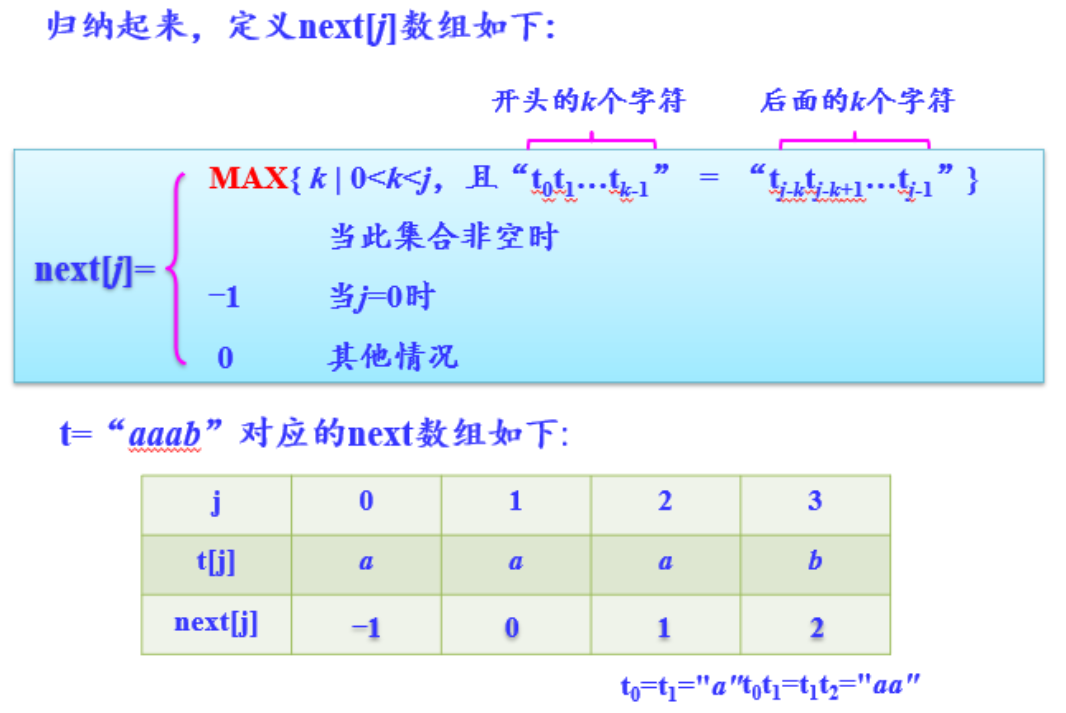### next数组的改进，nextval数组## 二叉树

• 定义：每个结点最多有两个子树的树结构。
• 性质：
1.在非空二叉树中，第i层的结点总数不超过 2^(i-1),(其中i>=1)；
2.深度为h的二叉树最多有(2^h)-1个结点(其中h>=1)，最少有h个结点；
3.对于任意一棵二叉树，如果其叶结点数为N0，而度数为2的结点总数为N2，则N0=N2+1
4.具有n个结点的完全二叉树的深度为（log₂n）+1
• 类型

### 1.完全二叉树：若设二叉树的高度为h，除第 h 层外，其它各层 (1～h-1) 的结点数都达到最大个数，第h层有叶子结点，并且叶子结点都是从左到右依次排布，这就是完全二叉树。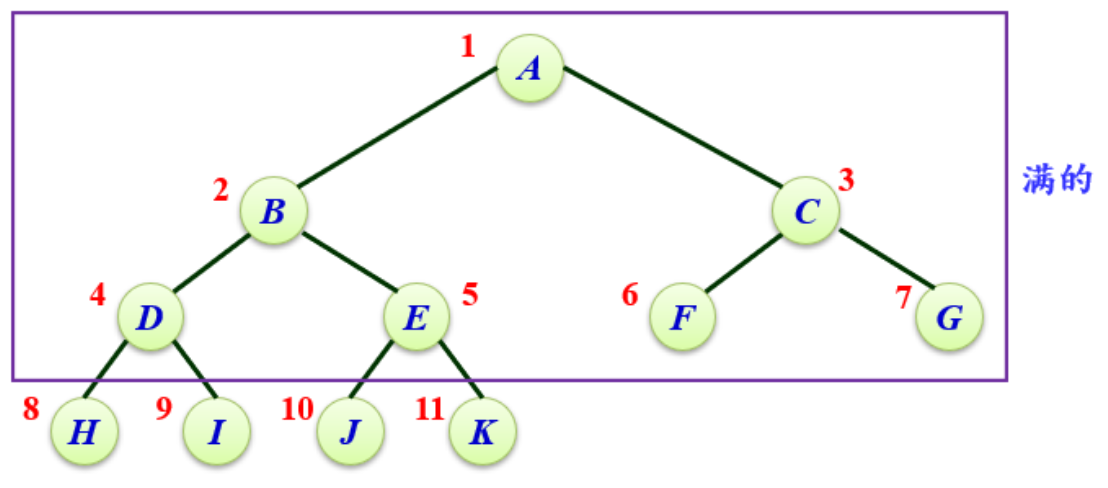### 2.满二叉树：除了叶结点外每一个结点都有左右子叶且叶子结点都处在最底层的二叉树。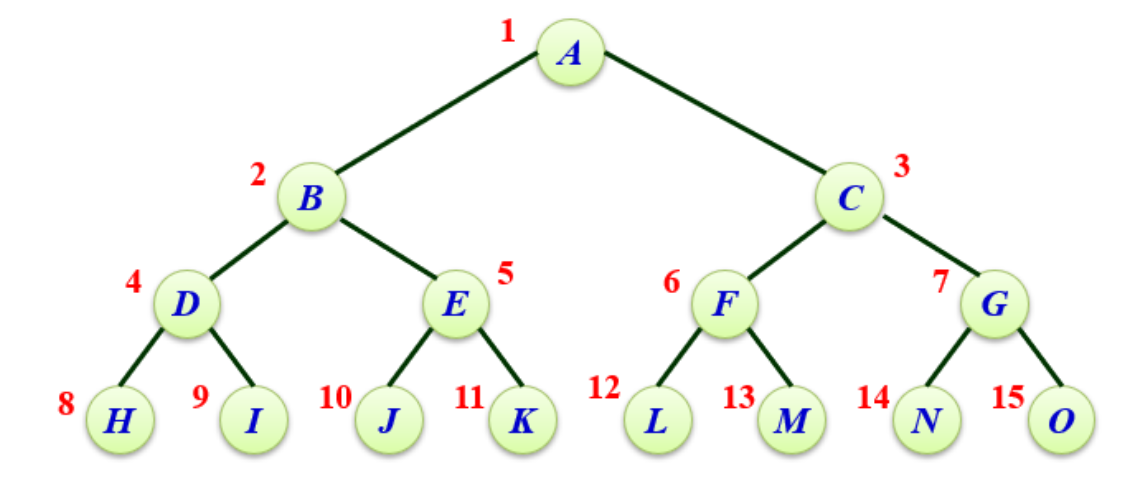### 完全二叉树的顺序储存结构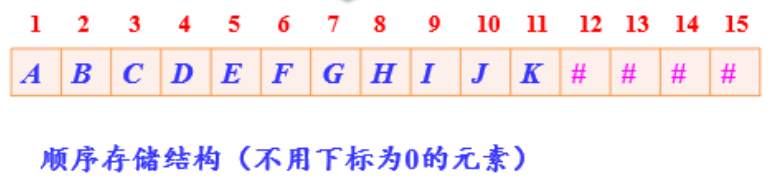### 非完全二叉树的顺序储存结构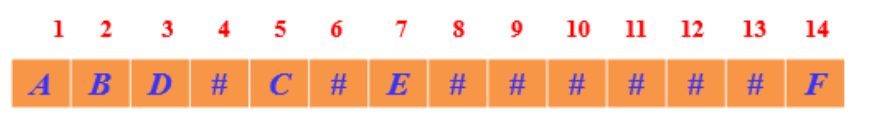### 特点：

1.各个结点的关系可以根据下标求出来，比如下标为i的结点，左孩子是2i，右孩子是2i+1，双亲是i/2
2.它用于存完全二叉树是很好的，这样可以最大限度地利用空间，不需要补齐那么多的空结点；但是如果遇到单分支很多的树，会浪费很多空间。

### 特点：

1.用指针对应关系，以上的定义便于寻找左右孩子，但是不好找双亲，当然，也可以加一个parent的指针，便于寻找双亲。结构体定义还是需要根据具体情况具体要求来定义。

typedef struct BiTNode {
char data;/*结点的数据*/
struct BiTNode* lchild, *rchild;/*左右孩子的指针*/
}BTNode,*BTree;


## 建树：

/*顺序存储结构转二叉链*/
typedef struct BiTNode
{
char data;
struct BiTNode* lchild, * rchild;    //指向左右孩子
}BTNode, * BTree;
BTree CreatTree(string s, int i) //顺序存储结构用字符串s表示
{
BTree bt;
bt = new BTNode;
int len;
len = s.size();    //字符串长度

if (i >len-1)    //超过长度返回NULL
{
return NULL;
}
if ( s[i] == '#')    //空结点返回NULL
{
return NULL;
}
/*根据左右孩子间i的关系递归建树*/
bt->data = s[i];
bt->lchild = CreatTree(s, 2 * i);
bt->rchild = CreatTree(s, 2 * i + 1);
return bt;
}

/*前序序列创建二叉树*/
BTree CreatBTree(string s,int &i)    //前序序列s，i为字符的下标
{
BTree bt;

if (i==s.size()||s[i] == '#')    //超过s长度或为空结点返回NULL
{
return NULL;
}
bt = new BNode;
/*前序遍历建树*/
bt->data = s[i];
bt->lchild = CreatBTree(s,++i);
bt->rchild = CreatBTree(s,++i);
return bt;
}

/*前序和中序序列创建二叉树*/
BTree CreatBTree(char* pre, char* in, int n) //前序序列用数组pre存储，中序序列用数组in存储
{
BTree bt;
int k;
char* p;

if (n <= 0) return NULL;
bt = new BTNode;
bt->data = *pre;    //根节点位置
for (p = in; p < in + n; p++)    //在中序序列中找到根节点位置
{
if (*p == *pre) break;
}
k = p - in;
bt->lchild = CreatBTree(pre + 1, in, k);
bt->rchild = CreatBTree(pre + k + 1, p + 1, n - k - 1);
return bt;
}

/*后序和中序序列创建二叉树*/
BTree CreatBTree(int* post, int* in, int n)    //后序序列用数组post存储，中序序列用数组in存储
{
BTree bt;
int k, * p;

if (n <= 0) return NULL;
bt = new BTNode;
bt->data = *(post + n - 1);    //后序序列末尾为根节点
for (p = in; p < in + n; p++)    //在中序序列中找到根节点
{
if (*p == *(post + n - 1)) break;
}
k = p - in;
bt->lchild = CreatBTree(post, in, k);
bt->rchild = CreatBTree(post + k, p + 1, n - k - 1);
return bt;
}


## 遍历

### 先序遍历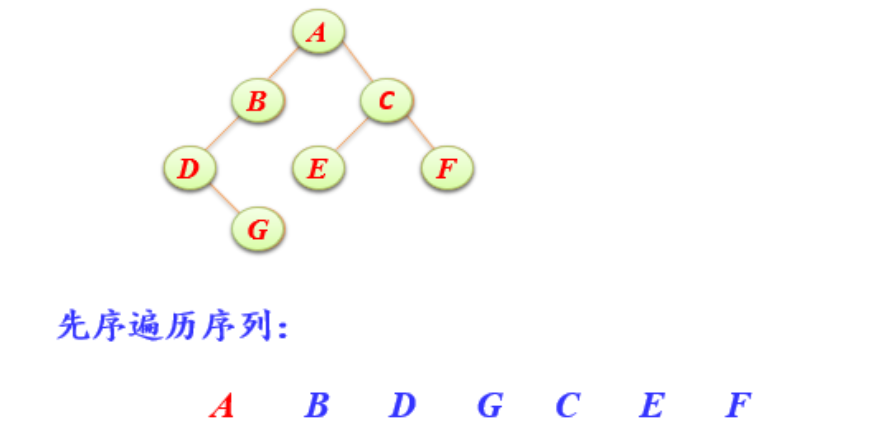void Preorder(BinTree BT)
{
if (BT==NULL) return;
cout>> BT->Data;
Preorder(BT->Left);
Preorder(BT->Right);
}


### 中序遍历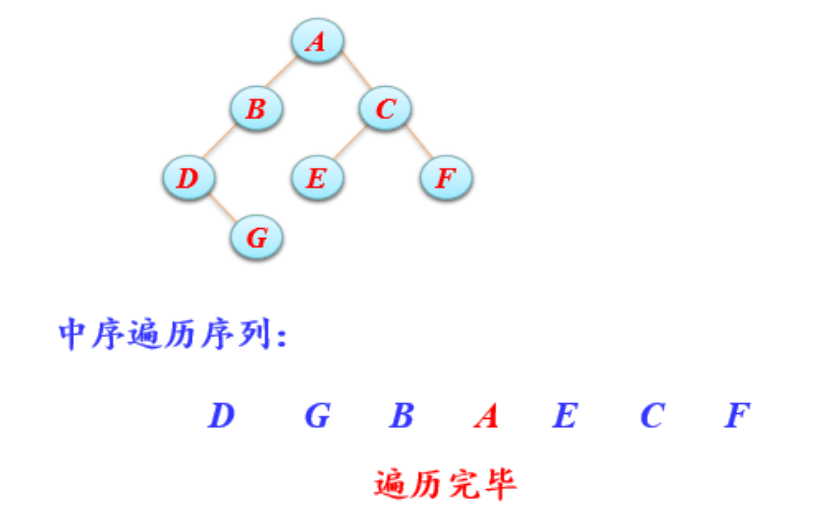void inorder(BinTree BT)
{
if (BT==NULL) return;
Preorder(BT->Left);
cout>> BT->Data;
Preorder(BT->Right);
}


### 后序遍历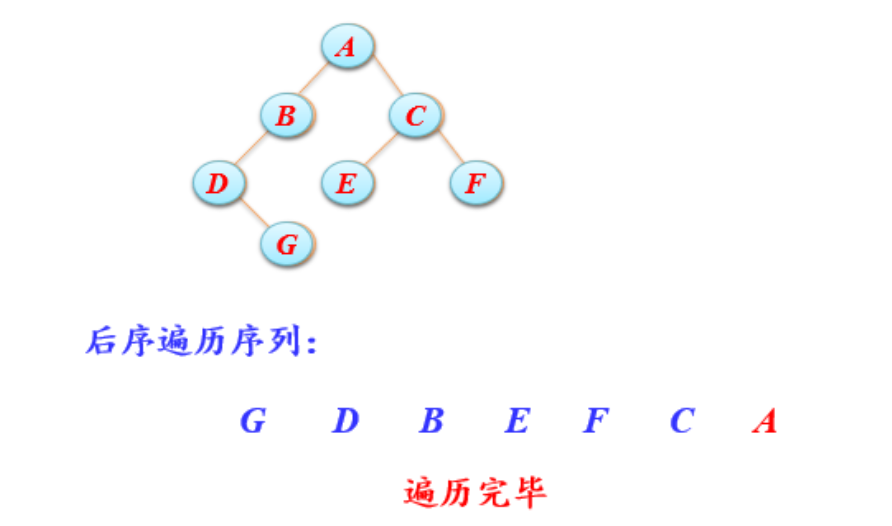void postorder(BinTree BT)
{
if (BT==NULL) return;
Preorder(BT->Left);
Preorder(BT->Right);
cout>> BT->Data;
}


### 层次遍历

void LevelOrder(BTree bt)
{
queue<BTree>q;     //使用队列遍历
BTree T;

q.push(bt);            //根节点入队
while (!q.empty())    //当队列不为空循环
{
T = q.front();
cout<< T->data ;
q.pop();           //出队一个结点，入队该结点的左右孩子
if (T->lchild)
{
q.push(T->lchild);
}
if (T->rchild)
{
q.push(T->rchild);
}
}
}


## 应用

### 求二叉树高度

int GetHeight(BinTree BT)
{
int h1,h2;
if (BT == NULL)
{
return 0;
}
h1 = GetHeight(BT->Left);
h2 = GetHeight(BT->Right);
if (h1 > h2)
{
return h1+1;
}
return h2+1;
}


## 树的结构、操作、遍历及应用

### 双亲存储结构：便于寻找双亲，不利于找孩子

 typedef struct
{　 ElemType data;/*结点数据*/
int parent;/*指向双亲*/
} PTree[MaxSize];


### 孩子链：孩子的数量不确定，可能大可能小，如果空结点过多，很浪费空间。便于找孩子，不利于找双亲.

typedef struct node
{      ElemType data;/*结点数据*/
struct node *sons[MaxSons];/*存孩子*/
}  TNode;


### 孩子兄弟链便于找孩子，不利于找父亲，但不会浪费太多空间。

typedef struct tnode
{      ElemType data;/*结点数据*/
struct tnode *son;/*指向孩子*/
struct tnode *brother;  2/*指向兄弟，同层的结点*/
} TNode;


## 线索二叉树

#### 记ptr指向二叉链表中的一个结点，以下是建立线索的规则：

（1）如果ptr->lchild为空，则存放指向中序遍历序列中该结点的前驱结点。这个结点称为ptr的中序前驱；
（2）如果ptr->rchild为空，则存放指向中序遍历序列中该结点的后继结点。这个结点称为ptr的中序后继；

### 显然，在决定lchild是指向左孩子还是前驱，rchild是指向右孩子还是后继，需要一个区分标志的。因此，我们在每个结点再增设两个标志域ltag和rtag，注意ltag和rtag只是区分0或1数字的布尔型变量，其占用内存空间要小于像lchild和rchild的指针变量。结点结构如下所示。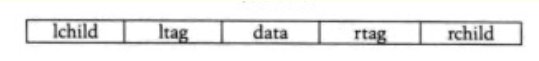#### 其中：

（1）ltag为0时指向该结点的左孩子，为1时指向该结点的前驱；
（2）rtag为0时指向该结点的右孩子，为1时指向该结点的后继；
（3）因此对于上图的二叉链表图可以修改为下图的样子。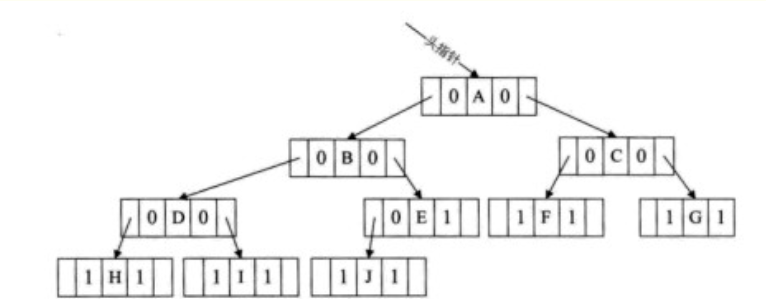### 定义：

typedef struct BiTNode {
char data;
int ltag ;/*标记是否有左孩子,0表示有孩子，1表示没有孩子（有线索）*/
int rtag;/*标记是否有右孩子*/
struct BiTNode* lchild, *rchild;
}BTNode,*BTree;


## 哈夫曼树和哈夫曼编码

### 结构特点：

typedef struct
{
int weight;//结点权重
int parent, left, right;//父结点、左孩子、右孩子在数组中的位置下标
}HTNode, *HuffmanTree;


### 实现代码：

/HT数组中存放的哈夫曼树，end表示HT数组中存放结点的最终位置，s1和s2传递的是HT数组中权重值最小的两个结点在数组中的位置
void Select(HuffmanTree HT, int end, int *s1, int *s2)
{
int min1, min2;
//遍历数组初始下标为 1
int i = 1;
//找到还没构建树的结点
while(HT[i].parent != 0 && i <= end){
i++;
}
min1 = HT[i].weight;
*s1 = i;

i++;
while(HT[i].parent != 0 && i <= end){
i++;
}
//对找到的两个结点比较大小，min2为大的，min1为小的
if(HT[i].weight < min1){
min2 = min1;
*s2 = *s1;
min1 = HT[i].weight;
*s1 = i;
}else{
min2 = HT[i].weight;
*s2 = i;
}
//两个结点和后续的所有未构建成树的结点做比较
for(int j=i+1; j <= end; j++)
{
//如果有父结点，直接跳过，进行下一个
if(HT[j].parent != 0){
continue;
}
//如果比最小的还小，将min2=min1，min1赋值新的结点的下标
if(HT[j].weight < min1){
min2 = min1;
min1 = HT[j].weight;
*s2 = *s1;
*s1 = j;
}
//如果介于两者之间，min2赋值为新的结点的位置下标
else if(HT[j].weight >= min1 && HT[j].weight < min2){
min2 = HT[j].weight;
*s2 = j;
}
}
}


## 并查集

### 结构体

typedef struct node
{
int data;		//结点对应人的编号
int rank;		//结点秩，大致为树的高度
int parent;		//结点对应双亲下标
} UFSTree;		//并查集树的结点类型


### 并查集初始化

void MAKE_SET(UFSTree t[]，int n)/*初始化并查集树*/
{
int i;/*循环计数*/
for (i = 1; i <= n; i++)
{
t[i].data = i;/*数据为该人的编号*/
t[i].rank = 0;/*秩初始化为0*/
t[i].parent = i;/*双亲初始化指向自已*/
}
}


### 两个元素各自所属的集合的合并

void UNION(UFSTree t[]，int x，int y)/*将x和y所在的子树合并*/
{
x = FIND_SET(t，x);/*查找x所在分离集合树的编号*/
y = FIND_SET(t，y);/*查找y所在分离集合树的编号*/
if (t[x].rank > t[y].rank)/*y结点的秩小于x结点的秩*/
{
t[y].parent = x; /*将y连到x结点上，x作为y的双亲结点*/
}
else/*y结点的秩大于等于x结点的秩*/
{
t[x].parent = y; /*将x连到y结点上，y作为x的双亲结点*/
if (t[x].rank == t[y].rank)/*x和y结点的秩相同*/
{
t[y].rank++;/*y结点的秩增1*/
}
}
}


# 2.阅读代码

## 2.1题目及解题代码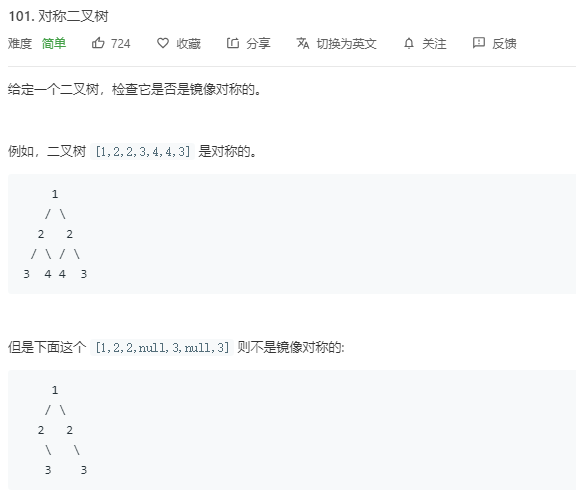class Solution {
public:
bool isSymmetric(TreeNode* root) {
if(!root) return true;

return __isSymmetric(root->left, root->right);
}
bool __isSymmetric(TreeNode* root1, TreeNode* root2){
bool flag1, flag2;
if(root1==nullptr){ // 两个子树都为空
if(root2!=nullptr) return false;
return true;
}
else if(root1!=nullptr){
if(root2==nullptr) return false;
// 两个子树都不为空
if(root1->val!=root2->val) return false;
flag1 = __isSymmetric(root1->left, root2->right);
flag2 = __isSymmetric(root1->right, root2->left);
}
return flag1&&flag2;
}
};


## 2.1.1 该题的设计思路

1) 两颗子树的根节点相同
2) 左子树的左子树和右子树的右子树对称（判断两棵树是否对称）
3) 右子树的左子树和左子树的右子树对称（判断两棵树是否对称）



## 2.1.2 该题的伪代码

 if两个子树都为空
return false;
else return true;
}
else if(root1不为空){
if(root2) return false;
if两个子树都不为空
return false;
递归



## 2.1.3 运行结果## 2.1.4分析该题目解题优势及难点

• 加强了对递归的应用
• 比较简单，明了，易懂，就是相较于递归遍历加上一些难度后的形式。

### 2.2 题目及解题代码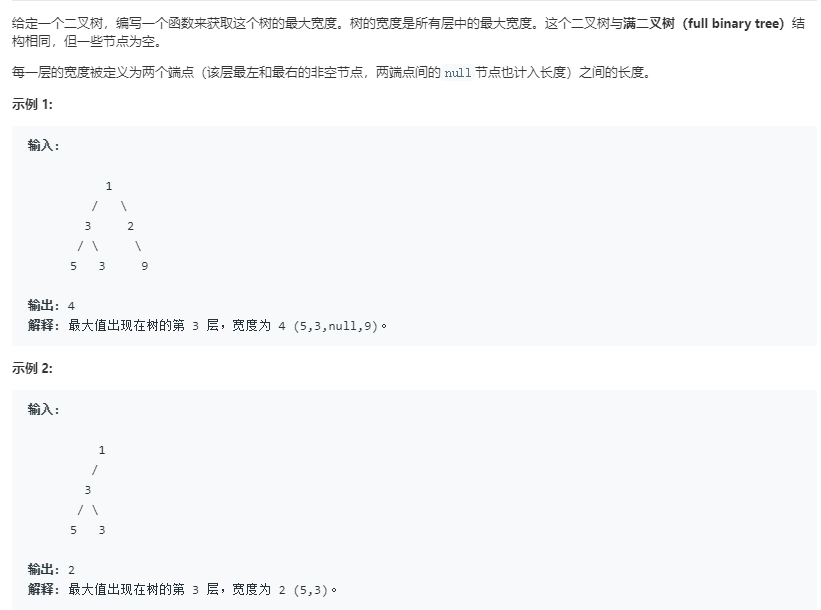int widthOfBinaryTree(treenode& root)
{

int ans = 0;
deque <Node> dque;  //定义一个空的队列
Node tmp = NULL;
if (root == NULL)
return ans;
dque.push_back(root);
while (!dque.empty())
{
while (!dque.empty() && dque.front() == NULL)
dque.pop_front();
while (!dque.empty() && dque.back() == NULL)
dque.pop_back();
int n = dque.size();
if (n == 0)
break;
if (ans < n)
n = ans;
for (int i = 0; i < n; i++)
{
tmp = dque.front();
dque.pop_front();
dque.push_back(tmp == NULL ? NULL : tmp->left);
dque.push_back(tmp == NULL ? NULL : tmp->right);
}
}
return ans;
}


## 2.2.1设计思路

• 借用队列运用层次遍历，找到每组最大值保存

## 2.2.2伪代码

int widthOfBinaryTree(TreeNode& root)
定义整型变量ans用来记录层宽度，并初始化为0
定义一个双端队列dque
定义一个空指针tmp
if root ==NULL do
返回ans值
end if  //即为空树的情况
否则root进队
while 队列不空 do
while 队列不空 and dque的队头为NULL do
将队头出队
end while
while 队列不空 and dque的对尾为NULL do
将队尾出队
end while
定义n存此时队列大小
将dque的size赋值给n
判断n和ans大小关系，将较大值赋给ans
if n=0 do  /*即此时队列为空*/
break
end if
for i=0 to n do i++
取队列头赋值给tmp
队列头出队
tmp的左右结点进队   //注意：null指针的左右两个儿子均为null。
end for
end while


## 2.2.3 运行结果## 2.2.4分析该题目解题优势及难点

• 优势：我们学过层次遍历，所以这题借助队列求值很容易可以看懂，且借助了vector容器，使代码更精简

## 2.3.1二叉树的最近公共祖先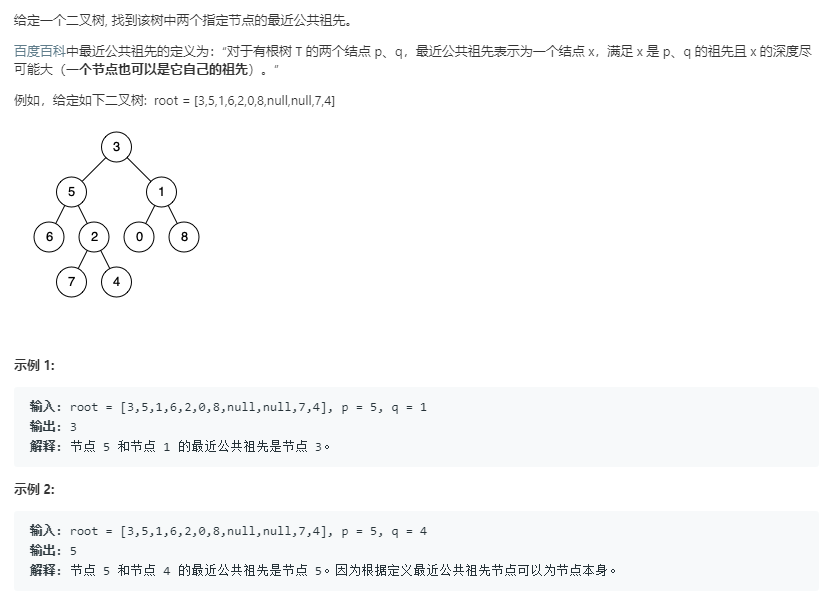class Solution {
public:
TreeNode* lowestCommonAncestor(TreeNode* root, TreeNode* p, TreeNode* q) {
if(root == NULL)return NULL;
if(p->val > root->val && q->val > root->val)
return lowestCommonAncestor(root->right, p, q);
else if(p->val < root->val && q->val < root->val)
return lowestCommonAncestor(root->left, p, q);
else return root;
}
};


## 2.3.2设计思路

• 如果p q的值都小于当前节点的值，则递归进入当前节点的左子树；
• 如果p q的值都大于当前节点的值，则递归进入当前节点的右子树；
• 如果当前节点的值在p q两个节点的值的中间，那么这两个节点的最近公共祖先则为当前的节点。

## 2.3.3伪代码

if(root为NULL||root==p||root==q)
return root;
end if

if(left && right)//说明在当前结点的左子树和右子树中分别找到p和q；
return root;//当前结点为最近祖先，返回当前结点；
end if
return left和right中不为空的结点；


## 2.3.4运行结果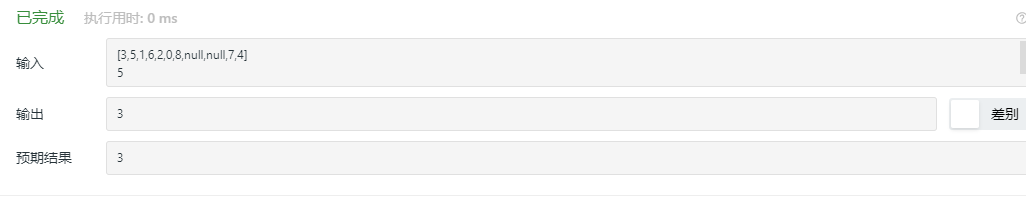## 2.3.5分析优势和难点

• 时间复杂度为O(log n)
• 这道题目的解法有许多种，这种算法是所有算法中代码量最少且比较容易实现的。
• 如果两个结点在同一子树时，我们会发现，只要找到一个就会直接递归回上一级，没有再继续往下遍历，这样我们就无法确定另一个节点是否存在。所以这种算法值局限于两个结点都存在在二叉树的情况。

## 2.4.1题目及代码class Solution {
public:
bool isCompleteTree(TreeNode* root) {
if(root == NULL){
return true;
}
queue<TreeNode*> q;
q.push(root);
bool isNULL = false;//之后的节点是否出行NULL
while(!q.empty()){
TreeNode* node = q.front();
q.pop();
if(node != NULL){
if(isNULL){
return false;
}
q.push(node->left);
q.push(node->right);
}
else{
isNULL = true;
}
}
return true;
}
};


## 2.4.3伪代码

class Solution {
public:
bool isCompleteTree(TreeNode* root){
queue<TreeNode*> q;
q.push(root);
bool类型为了判断之后的节点是否为NULL
while(队非空)
if(node != NULL)
if(isNULL)
return false;
end if;
左右孩子入队；
end if;
else
isNULL = true;
end else;
end while;
return true;
}
};


## 2.4.4运行结果## 2.4.5分析优势和难点

• 难点在于isNULL的设定上，对于末尾NULL的处理。
• 时间复杂度：O（n）
• 空间复杂度：O（n）
posted on 2020-04-12 21:44  洪志鸿  阅读(278)  评论(0编辑  收藏  举报